# Automata, homomorphism of

(diff) ← Older revision | Latest revision (diff) | Newer revision → (diff)

A mapping of the input and output alphabets and of the set of states of an automaton into analogous sets of a second automaton that preserves the transition and output functions. More precisely, a homomorphism of an automaton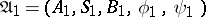into an automaton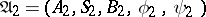(cf. Automaton, finite) is a mapping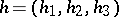of the set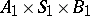into the set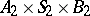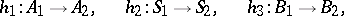such that the following equalities are valid for anyfromand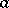from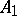: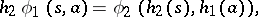Initialized automata are subject to the additional requirement that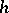maps the initial state to the initial state. The automataand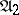are said to be homomorphic if there exists a homomorphismof automata mappinginto. If, in addition,is one-to-one,is called an isomorphism, and the automataandare said to be isomorphic automata. If the alphabetsand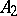, and also the alphabets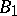and, are identical and the mappings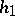and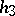are the identity mappings, the homomorphism (isomorphism)is known as a state homomorphism (state isomorphism). Input and output homomorphisms (isomorphisms) are defined in a similar manner. State-isomorphic automata and state-homomorphic initialized automata are equivalent (cf. Automata, equivalence of).

The concept of a homomorphism of automata is used in the context of problems concerning minimization, decomposition and completeness of automata, among others.

How to Cite This Entry:
Automata, homomorphism of. Encyclopedia of Mathematics. URL: http://encyclopediaofmath.org/index.php?title=Automata,_homomorphism_of&oldid=12330
This article was adapted from an original article by A.A. Letichevskii (originator), which appeared in Encyclopedia of Mathematics - ISBN 1402006098. See original article